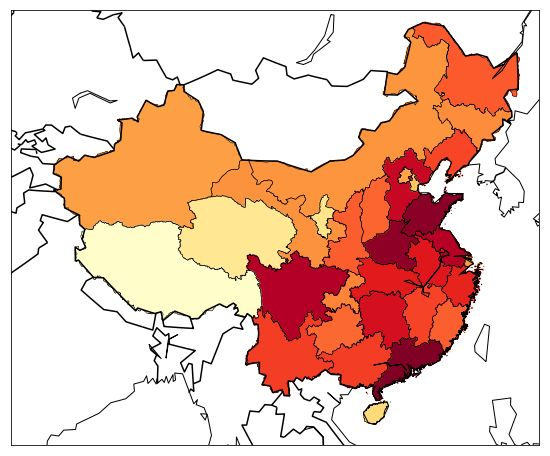# 用Python画中国地图（二）## 上色

``````    from matplotlib.patches import Polygon

ax = plt.gca()
for nshape, seg in enumerate(m.states):
poly = Polygon(seg, facecolor='r')
````````````    m.readshapefile('TWN_adm_shp/TWN_adm0', 'taiwan', drawbounds=True)
for nshape, seg in enumerate(m.taiwan):
poly = Polygon(seg, facecolor='r')
````````````    for shapedict in m.states_info:
statename = shapedict['NL_NAME_1']
p = statename.split('|')
if len(p) > 1:
s = p
else:
s = p
print(s)
for shapedict in m.taiwan_info:
s = shapedict['NAME_CHINE']
print(s)
``````

``````    安徽
北京
重庆
福建
福建
福建
...
``````

## 数据

``````    df = pd.read_csv('chnpop.csv')
``````## 渲染

``````    cmap = plt.cm.YlOrRd
``````

``````    colors[s] = cmap(np.sqrt((pop - vmin) / (vmax - vmin)))[:3]
``````

``````    color = rgb2hex(colors[statenames[nshape]])
poly = Polygon(seg, facecolor=color, edgecolor=color)
````````````import matplotlib.pyplot as plt
from mpl_toolkits.basemap import Basemap
from matplotlib.patches import Polygon
from matplotlib.colors import rgb2hex

plt.figure(figsize=(16,8))
m = Basemap(
llcrnrlon=77,
llcrnrlat=14,
urcrnrlon=140,
urcrnrlat=51,
projection='lcc',
lat_1=33,
lat_2=45,
lon_0=100
)
m.drawcountries(linewidth=1.5)
m.drawcoastlines()

df['省名'] = df.地区.str[:2]
df.set_index('省名', inplace=True)

statenames=[]
colors={}
cmap = plt.cm.YlOrRd
vmax = 100000000
vmin = 3000000
for shapedict in m.states_info:
statename = shapedict['NL_NAME_1']
p = statename.split('|')
if len(p) > 1:
s = p
else:
s = p
s = s[:2]
if s == '黑龍':
s = '黑龙'
statenames.append(s)
pop = df['人口数'][s]
colors[s] = cmap(np.sqrt((pop - vmin) / (vmax - vmin)))[:3]

ax = plt.gca()
for nshape, seg in enumerate(m.states):
color = rgb2hex(colors[statenames[nshape]])
poly = Polygon(seg, facecolor=color, edgecolor=color)